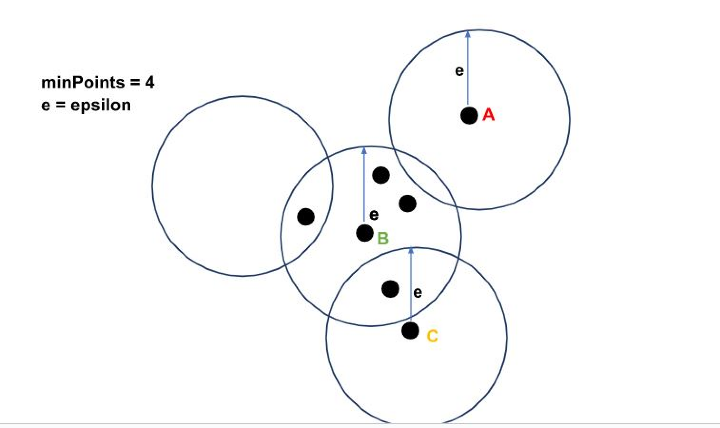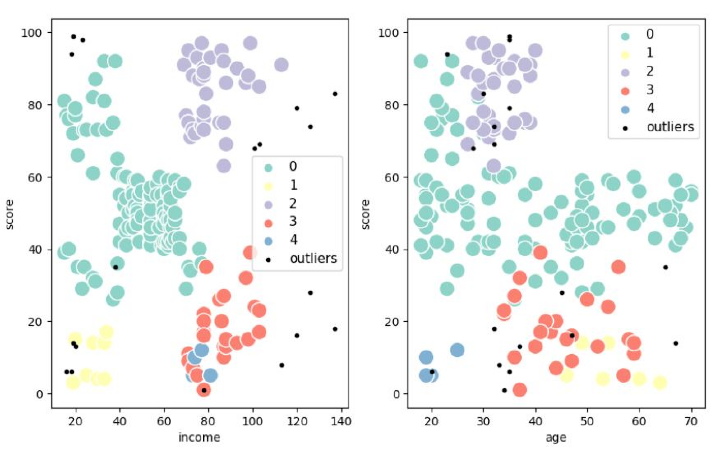# DBSCAN Clustering in ML | Density based clustering

## Introduction

DBSCAN is the abbreviation for Density-Based Spatial Clustering of Applications with Noise. It is an unsupervised clustering algorithm.DBSCAN clustering can work with clusters of any size from huge amounts of data and can work with datasets containing a significant amount of noise. It is basically based on the criteria of a minimum number of points within a region.

## What is DBSCAN Algorithm?

DBSCAN algorithm can cluster densely grouped points efficiently into one cluster. It can identify local density in the data points among large datasets. DBSCAN can very effectively handle outliers. An advantage of DBSACN over the K-means algorithm is that the number of centroids need not be known beforehand in the case of DBSCAN.

DBSCAN algorithm depends upon two parameters epsilon and minPoints.

Epsilon is defined as the radius of each data point around which the density is considered.

minPoints is the number of points required within the radius so that the data point becomes a core point.

The circle can be extended to higher dimensions.

## Working of DBSCAN Algorithm

In the DBSCAN algorithm, a circle with a radius epsilon is drawn around each data point and the data point is classified into Core Point, Border Point, or Noise Point. The data point is classified as a core point if it has minPoints number of data points with epsilon radius. If it has points less than minPoints it is known as Border Point and if there are no points inside epsilon radius it is considered a Noise Point.

Let us understand working through an example.In the above figure, we can see that point A has no points inside epsilon(e) radius. Hence it is a Noise Point. Point B has minPoints(=4) number of points with epsilon e radius , thus it is a Core Point. While the point has only 1 ( less than minPoints) point, hence it is a Border Point.

### Steps Involved in DBSCAN Algorithm.

• First, all the points within epsilon radius are found and the core points are identified with number of points greater than or equal to minPoints.

• Next, for each core point, if not assigned to a particular cluster, a new cluster is created for it.

• All the densely connected points related to the core point are found and assigned to the same cluster. Two points are called densely connected points if they have a neighbor point that has both the points within epsilon distance.

• Then all the points in the data are iterated, and the points that do not belong to any cluster are marked as noise.

### Code Implementation

## DBSCAN

import numpy as np
import pandas as pd
import matplotlib.pyplot as plt
from sklearn.cluster import DBSCAN
import seaborn as sns

data.rename(columns={'CustomerID':'customer_id','Gender':'gender','Age':'age','Annual Income (k\$)':'income','Spending Score (1-100)':'score'},inplace=True)
features = ['age', 'income', 'score']
train_x = data[features]
cls = DBSCAN(eps=12.5, min_samples=4).fit(train_x)
datasetDBSCAN = train_x.copy()
datasetDBSCAN.loc[:,'cluster'] = cls.labels_
datasetDBSCAN.cluster.value_counts().to_frame()

outliers = datasetDBSCAN[datasetDBSCAN['cluster']==-1]

fig, (ax) = plt.subplots(1,2,figsize=(10,6))

sns.scatterplot(x='income', y='score',data=datasetDBSCAN[datasetDBSCAN['cluster']!=-1],hue='cluster', ax=ax, palette='Set3', legend='full', s=180)

sns.scatterplot(x='age', y='score',

data=datasetDBSCAN[datasetDBSCAN['cluster']!=-1],

hue='cluster', palette='Set3', ax=ax, legend='full', s=180)

ax.scatter(outliers['income'], outliers['score'], s=9, label='outliers', c="k")

ax.scatter(outliers['age'], outliers['score'], s=9, label='outliers', c="k")
ax.legend()
ax.legend()

plt.setp(ax.get_legend().get_texts(), fontsize='11')
plt.setp(ax.get_legend().get_texts(), fontsize='11')

plt.show()


### Output## Advantages of the DBSCAN Algorithm

• DBSCAN does not require the number of centroids to be known beforehand as in the case with the K-Means Algorithm.

• It can find clusters with any shape.

• It can also locate clusters that are not connected to any other group or clusters. It can work well with noisy clusters.

• It is robust to outliers.

## Disadvantages of the DBSCAN Algorithm

• It does not work with datasets that have varying densities.

• Cannot be employed with multiprocessing as it cannot be partitioned.

• Cannot find the right cluster if the dataset is sparse.

• It is sensitive to parameters epsilon and minPoints

## Applications of DBSCAN

• It is used in satellite imagery.

• Used in XRay crystallography

• Anamoly detection in temperature.

## Conclusion

DBSCAN is an unsupervised clustering technique that performs better than other clustering algorithms in the case of outliers and arbitrarily shaped clusters.DBSCAN clusters together regions that are dense based on distance measurement. It is a spatial clustering algorithm that can work extremely well with noise data as well.

Updated on: 22-Sep-2023

277 Views